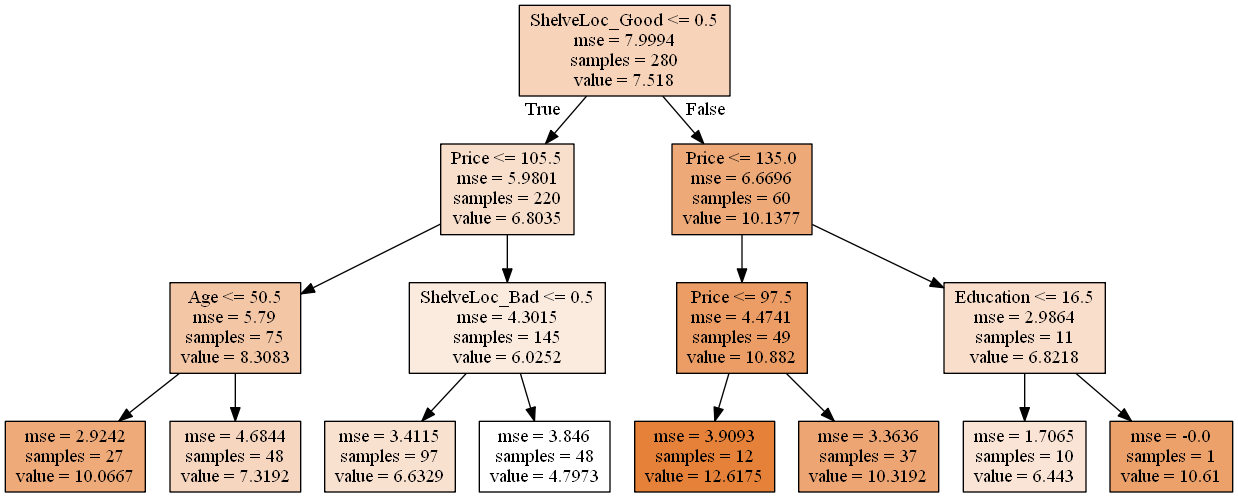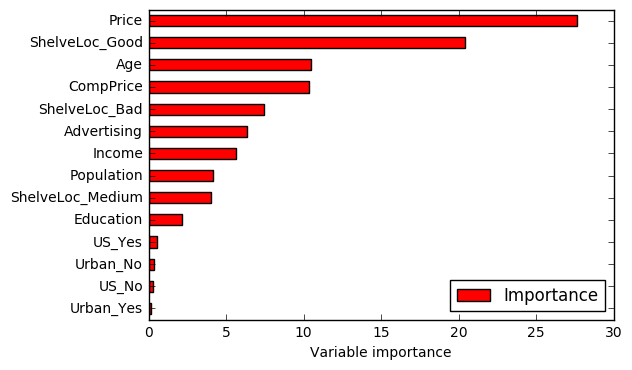# Exercise 8.8

import pandas as pd
import numpy as np
import pydotplus  # Check the references if you need help to install this module.
import matplotlib.pyplot as plt

from sklearn.model_selection import train_test_split
from IPython.display import Image  # To plot decision tree.
from sklearn.externals.six import StringIO  # To plot decision tree.
from sklearn.metrics import mean_squared_error
from sklearn.model_selection import GridSearchCV
from sklearn.ensemble import BaggingRegressor
from sklearn.ensemble import RandomForestRegressor

%matplotlib inline

df = pd.read_csv('../data/Carseats.csv')

df.head()

Sales CompPrice Income Advertising Population Price ShelveLoc Age Education Urban US
0 9.50 138 73 11 276 120 Bad 42 17 Yes Yes
1 11.22 111 48 16 260 83 Good 65 10 Yes Yes
2 10.06 113 35 10 269 80 Medium 59 12 Yes Yes
3 7.40 117 100 4 466 97 Medium 55 14 Yes Yes
4 4.15 141 64 3 340 128 Bad 38 13 Yes No
# Dummy variables
# Transform qualitative variables into quantitative to enable the use of regressors.
df = pd.get_dummies(df)

df.head()

Sales CompPrice Income Advertising Population Price Age Education ShelveLoc_Bad ShelveLoc_Good ShelveLoc_Medium Urban_No Urban_Yes US_No US_Yes
0 9.50 138 73 11 276 120 42 17 1.0 0.0 0.0 0.0 1.0 0.0 1.0
1 11.22 111 48 16 260 83 65 10 0.0 1.0 0.0 0.0 1.0 0.0 1.0
2 10.06 113 35 10 269 80 59 12 0.0 0.0 1.0 0.0 1.0 0.0 1.0
3 7.40 117 100 4 466 97 55 14 0.0 0.0 1.0 0.0 1.0 0.0 1.0
4 4.15 141 64 3 340 128 38 13 1.0 0.0 0.0 0.0 1.0 1.0 0.0
# This function creates images of tree models using pydot
# Source: http://nbviewer.jupyter.org/github/JWarmenhoven/ISL-python/blob/master/Notebooks/Chapter%208.ipynb
# The original code used pydot instead of pydotplus. We didn't change anything else.
def print_tree(estimator, features, class_names=None, filled=True):
tree = estimator
names = features
color = filled
classn = class_names

dot_data = StringIO()
export_graphviz(estimator, out_file=dot_data, feature_names=features, class_names=classn, filled=filled)
graph = pydotplus.graph_from_dot_data(dot_data.getvalue())
return(graph)


# (a)

# Split data into training and test set
X = df.ix[:,1:]
y = df['Sales']

X_train, X_test, y_train, y_test = train_test_split(X, y, test_size=.3, random_state=1)


# (b)

# Fit regression tree
rgr = DecisionTreeRegressor(max_depth=3)  # We could have chosen another max_depth value.
rgr.fit(X_train, y_train)

DecisionTreeRegressor(criterion='mse', max_depth=3, max_features=None,
max_leaf_nodes=None, min_impurity_split=1e-07,
min_samples_leaf=1, min_samples_split=2,
min_weight_fraction_leaf=0.0, presort=False, random_state=None,
splitter='best')

# Plot the tree
graph = print_tree(rgr, features=list(X_train.columns.values))
Image(graph.create_png())Interpretation: According to the tree, ShelveLoc is the most important factor in determining Sales. Shelveloc is an indicator of the quality of the shelving location — that is, the space within a store in which the car seat is displayed — at each location. Cars with a good shelving location sell more (value = 10.1377) than cars in bad or medium shelving locations (value = 6.8035). Among each of these groups, Price is the second most important factor in determining Sales. For example, for cars with a good shelving location, when the Price is above 135, Sales decrease (6.8218 vs. 10.882). The same analysis logic applies for the remaining branches of the regression tree.

# Test MSE
print('Test MSE: ', mean_squared_error(y_test, rgr.predict(X_test)))

Test MSE:  4.72516600994


# (c)

To determine the optimal level of tree complexity using cross-validation we will use GridSearchCV.

# Build a regressor
rgr = DecisionTreeRegressor(random_state=1)

# Grid of parameters to hypertune
param_grid = {'max_depth':[1,2,3,4,5,6,7,8,9,10]}

# Run grid search
grid_search = GridSearchCV(rgr,
param_grid=param_grid,
cv=5)
grid_search.fit(X_train, y_train)

GridSearchCV(cv=5, error_score='raise',
estimator=DecisionTreeRegressor(criterion='mse', max_depth=None, max_features=None,
max_leaf_nodes=None, min_impurity_split=1e-07,
min_samples_leaf=1, min_samples_split=2,
min_weight_fraction_leaf=0.0, presort=False, random_state=1,
splitter='best'),
fit_params={}, iid=True, n_jobs=1,
param_grid={'max_depth': [1, 2, 3, 4, 5, 6, 7, 8, 9, 10]},
pre_dispatch='2*n_jobs', refit=True, return_train_score=True,
scoring=None, verbose=0)

# Find the best estimator
grid_search.best_estimator_

DecisionTreeRegressor(criterion='mse', max_depth=5, max_features=None,
max_leaf_nodes=None, min_impurity_split=1e-07,
min_samples_leaf=1, min_samples_split=2,
min_weight_fraction_leaf=0.0, presort=False, random_state=1,
splitter='best')


The best value for max_depth using cross-validation is 5.

Note: Pruning is currently not supported by scikit-learn, so we didn't solve that part of the exercise.

# (d)

# Fit bagging regressor
rgr = BaggingRegressor()
rgr.fit(X_train, y_train)

BaggingRegressor(base_estimator=None, bootstrap=True,
bootstrap_features=False, max_features=1.0, max_samples=1.0,
n_estimators=10, n_jobs=1, oob_score=False, random_state=None,
verbose=0, warm_start=False)

# Test MSE
print('Test MSE:', mean_squared_error(y_test, rgr.predict(X_test)))

Test MSE: 3.33869740833


Note: We didn't find a way to get variable's importance using scikit-learn, so we didn't solve that part of the exercise.

# (e)

# Fit random forest regressor
rgr = RandomForestRegressor()
rgr.fit(X_train, y_train)

RandomForestRegressor(bootstrap=True, criterion='mse', max_depth=None,
max_features='auto', max_leaf_nodes=None,
min_impurity_split=1e-07, min_samples_leaf=1,
min_samples_split=2, min_weight_fraction_leaf=0.0,
n_estimators=10, n_jobs=1, oob_score=False, random_state=None,
verbose=0, warm_start=False)

# Test MSE
print('Test MSE:', mean_squared_error(y_test, rgr.predict(X_test)))

Test MSE: 3.23118576667

# Variable importance
importance = pd.DataFrame({'Importance':rgr.feature_importances_*100}, index=X_train.columns)
importance.sort_values('Importance', axis=0, ascending=True).plot(kind='barh', color='r')
plt.xlabel('Variable importance')
plt.legend(loc='lower right')

<matplotlib.legend.Legend at 0xec02978>The test MSE decreased when compared with the previous answers. One possible explanation for this result is the effect of m. In random forests, the number of variables considered at each split changes. This means that, at each split, only a subset of predictors is taken into account. In some sense, this works as a process to decorrelate the decision trees. For example, in baggin, if there is a strong predictor in the data set, most of the bagged trees wil use this predictor in the top split. As a consequence, the predictions from the bagged trees will tend to be highly correlated. In random forests, this doesn't happen because the method forces each split to consider only a subset of the predictors. Hence, the method leads to models with reducted variance and increased reliability. That's a possible reason for the reduction verified in the test MSE value.

• https://packaging.python.org/installing/ (help to install Python modules)